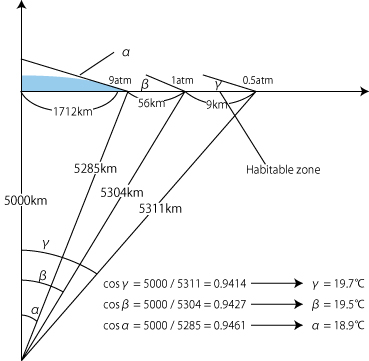ページ内のクイックリンク
HOME  >  english  >  Cubic Earth  >  Vertical Distribution of the Atmospheric Temperature

Cubic Earth

# Vertical Distribution of the Atmospheric Temperature

## Factors that Decides Atmospheric TemperatureFig. 4 Relationship between atmospheric pressure and inclination of the land

Temperatures decrease with altitudes as you experience in the mountaineering. The decreasing rate is 6.5℃/km. The surface temperatures also vary within one day as well as the seasonal cycle. There are two factors which control the atmospheric temperature.

They are:
1. The amount of solar energy given to the Earth
2. The absorption rate of the Earth for the solar radiation. 30% of the solar radiation returns to the space by reflection due to clouds and ice sheets at poles. Therefore, 70% of the solar radiation given to the Earth is used to heat the atmosphere. We call this fact as the albedo of the Earth is 0.3.
3. The amount of the greenhouse gas (majority is water vapor)

There are large variations of the atmospheric temperature, depending on regions and time on the surface of the real Earth. However, if you observe the global average of the Earth temperatures from the space, you will find that the Earth temperature is always -18℃. This temperature corresponds to the average temperature at 5 km high from the sea surface. The global average of the surface temperature is about 15℃.

## Temperature on Sea Surface of Cubic Earth will be high

The atmospheric environment of the cubic Earth over the ocean is similar with that of the real Earth. Let us assume that the atmospheric temperature of the cubic Earth is -18℃. Then, the temperature at 1atm is 15℃, because the temperature lapse rate is 6.5℃/km. If the atmosphere of the cubic Earth has the same lapse rate, the temperature at the sea level of the cubic Earth is 73.5℃. The temperature at the sea level of the cubic Earth is supposed to be higher than that of the real Earth.

The planetary temperature depends on albedo as described above. The albedo depends on cloud amount. It increases with cloud amount. The cloud amount of the real Earth is always about 0.5 and it yields 0.3 of the albedo.
We assume that the temperature at the sea level on the cubic Earth is 65℃. This assumption means that we assume that the cloud amount in the cubic Earth is greater than that in the real Earth.

このページのトップへ